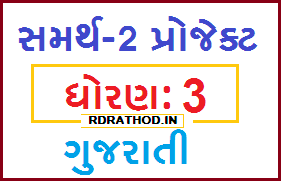-->

# STD 3 Gujarati Samarth 2 Project for Online Shikshak Talim | Useful for All Gujarati Teachers

Gujarati Samarth 2 Online Teachers Training Project for std 3. Gujarati Subject na Shikshako Mate Samarth 2 ni Talim Mate Upayogi Gujarati Subject na Project no namuno Download karo pdf.

Subject : Gujarati
Standard : 3
Project Name : Chalo Pakshio ne Janie
Unit : Sinh Ane UndarSTD 3 Gujarati Samarth 2 Project

1. This project is designed to achieve achievement / achievement in children? : Compares heavy and light objects with the use of plain scales.

2. Project no Dhey: Aim: Children experience a lot of difficulty and difficulty in adding and subtracting decimal numbers. In the present form, children can easily add and subtract decimal numbers through this activity, especially by setting the teenager to decimal as well as subtracting the decimal. Detailed description of the activity to be added to keep the digits on the right as well as on the left: Work has been done by creating four groups of squares for which cards are already prepared, in which one hundred tens of units will be given the sum of decimal fractions, the sum of the decimal fractions in each group, and the amount of the subtraction given to me. Easily exemplified in this activity, children often make mistakes in putting decimal china yes below decimal china yes. The first is calculated by placing the same amount in this card. Subtracting the decimal power from the decimal for the sum as well as the sum from the ascending unit from the decimal as well as the subtraction and addition of others of this type, and so on. This activity is done as well as the left side as well as the right side are required

3. Detailed description of the activity: Here subtracting the decimal power from the decimal for the sum of gold as well as the sum of the subtasks from the unit as well as the subtraction of the others as well as the sum is added as well. This activity is done. For example 0. 53 + 0. 42 = 0. 77 Thus children can easily evaluate the actions taken in simple sums as well as subtraction, and the result is the amount given to the children by this activity. If the need for tens is to be deducted from the next digit, then if the sum is double digits, then add Putting the digits in the decimal digits puts the sum of the digits on the front digits. This activity shows a 70% to 80% improvement in the mistakes that the children make. Once the child has started putting money on the card, he does not even need the card. Like the rest, the sum of decimals can be subtracted

4. Mulyankan Ane Parinam (Assessment and Outcome) : After doing this activity, children were asked to give different items in the office. If a child gives a pencil, a child gives rubber. If a child gives a puppy, a child gives a pavement. If a child gives a Minya color, a child gives a ballpen. They were asked to take either of these two items to see which of them was tilted. So the children started to say that the pencil and the rubber ball were tilted. So the teacher asked who would be the heavier of these two? So the bulk replied that the rubber would be heavier. And if the two of them were light, the child replied that the pencil would be light. In this way, every child had such activity and children were compared.

5. Chintan (Meditation): This activity will put the children in the cards given to them by following the pattern given to them below, but they will be able to overcome their problem as slowly as they do the work of their peers. So that even the dear children can be solved with this activity as well as solve and subtract questions So the function that doesn't work from k can be through this activity. Once a child is allowed to put money according to the card, they can add and subtract decimals in the form of a simple sum and subtraction.